Optics
Volume 4, Issue 3-1, June 2015, Pages: 1-4

Residual Stress Measurement of an Interference Fit Specimen Based on Moiré Interferometry and Hole-Drilling Method

Baoge Zhang, Jianguo Zhu*

Faculty of Civil Engineering and Mechanics, Jiangsu University, Zhenjiang, China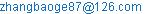(Baoge Zhang)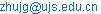(Jianguo Zhu)

Baoge Zhang, Jianguo Zhu. Residual Stress Measurement of an Interference Fit Specimen Based on Moiré Interferometry and Hole-Drilling Method. Optics. Special Issue: Optical Techniques for Deformation, Structure and Shape Evaluation. Vol. 4, No. 3-1, 2015, pp. 1-4. doi: 10.11648/j.optics.s.2015040301.11

Abstract: In this paper, moiré interferometry is combined with hole-drilling method to measure the residual stress of an interference fit specimen. And, finite element method is used to calibrate the coefficient of hole-drilling method. The experimental results are consistent with theoretical results, which manifests that moiré interferometry combined with hole-drilling method are reliable and effective for residual stress measurement.

Keywords: Moiré Interferometry, Hole-Drilling Method, Interference Fit, Residual Stress

1. Introduction

Residual stress is the stress which remains in a body and balances by itself without any external forces and temperature fields. Residual stress widely exists in bodies of metal, wood, and, organic materials when there is nonuniform deformation in the internal of the materials. Residual stress influences the static strength, corrosion resistant, and fatigue resistant of component remarkably, and it can be detrimental to the performance of a material and the life of a component [1-3]. Alternatively, beneficial residual stress can be introduced deliberately [4-6]. And it is of great importance to measure residual stress effectively.

There are many methods to measure residual stress, and it can be divided into destructive, and non-destructive methods depend on whether the specimen is damaged . Hole-drilling method is considered to be the most mature and widely employed destructive method , because of its high precision of measurement, small damage to specimens, and low cost.

As for the traditional hole-drilling method, the released deformation is usually measured by strain gauges, and then, the stress is calculated by the formula between stress and strain. However, the strain gauge has its limitations, for example, it can only measure the local strain, and the measurement’s accuracy depends on the align accuracy of drilling.

Moiré interferometry was proposed at the end of the 19th century, and was developed to measure in-plane displacements of essentially flat surfaces. It is a non-contact and real-time measurement technique with features of high sensitivity, large range, and excellent quality of stripe. At present, the investigation on moiré interferometry has reached to a maturity stage, and the method is widely used in the materials science, nondestructive testing, fracture mechanics, and, mesoscopic mechanics.

Moiré interferometry can be combined with hole-drilling method for residual stress measurement. Instead of local strain measurement, the whole field of displacement is obtained by analysis of fringe patterns. Hole-drilling method assumes that a plate is isotropic and subjected by in-plane stresses, and the distribution of stresses in the internal of the plate changes after a hole is drilled. The relationship between the radial displacement and residual stress caused by drilling is given by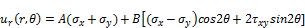(1)

where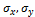represent the stress component in the x and y direction, respectively, and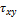represents the shear stress component in Cartesian coordinate system,represents the radius, and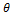represents the polar angle, A and B represent the coefficients, respectively. When a hole is drilled in the plate, the stress condition is given as follows,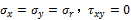, and as the result of the axisymmetry of its boundary and stress, Eq. (1) is simplified as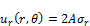(2)

LY12 aluminum alloy is widely used in aerospace and other industries, and it is of great importance to study the residual stress in the component of the material.

2. Moiré Interferometry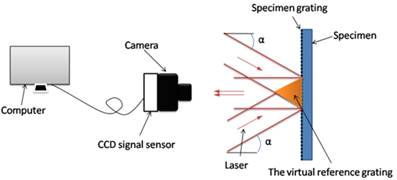Figure 1. Principle of moiré interferometry

Fig.1 presents the principle of moiré interferometry. When two laser beams are irradiated symmetrically on a specimen grating, a virtual grating, called the reference grating, with twice frequency of the specimen grating, is produced in the cross area of the two laser beams. Then, the specimen grating and the reference grating interfere mutually, and produce moiré fringes when the specimen’s deformation occurs. The stripes on the moiré fringe represent the contour lines of in-plane displacement, and the formula of the displacement in the x and y axes of every stripe is given by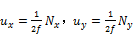(3)

where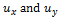represent the displacement in the x and y axes, respectively, f represents the frequency of the specimen grating,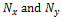represent the fringe orders in the x and y axes, respectively.

3. Specimen Preparation and Experiments

The interference fit specimen is widely used in mechanical engineering to pass torque and axial force. In the present study, the material of the interference fit specimen is aluminum alloy LY12, and its geometric and material properties are given in Table 1. In this paper, a sleeve and a plate were used to assemble the interference fit specimen by heating the sleeve and cooling the plate, which is shown in Fig.2.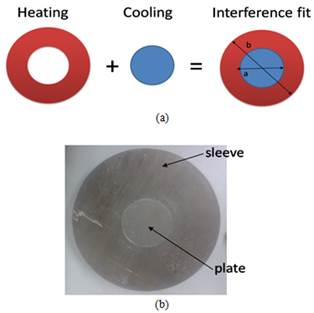Figure 2. (a) Assembly process of the interference fit specimen and (b) The interference fit specimen.

Table 1. The geometric and material properties of the interference fit specimen

 Parameter Plate sleeve Radius a/25.034mm b/75mm  0.068mm Height/H 10mm Elastic modulus/E 73GPa Poisson's ratio/u 0.3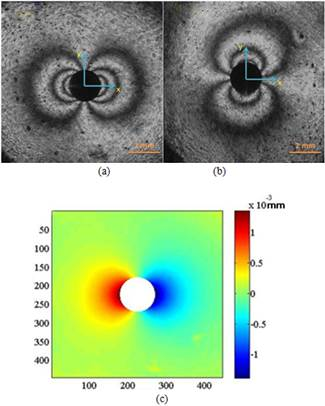Figure 3. (a) Moiré fringes of U field, (b) Moiré fringes of V field, and (c) U field of the displacement

During the experiment, first, a grating of 1200 lines/mm was replicated on the surface of the plate. Second, a drill with a diameter of 2 mm was used, and the depth of the hole was 1.89 mm. Third, the specimen was put on the moiré interferometer to record the moiré fringes, which is shown in Figs.3 (a) and (b), and it can be seen that there were three stripes in the U field and V field, which means that the stress magnitude on x axis and y axis were approximately equal, and it matched to the theoretical solution of the interference fit specimen. Besides, the stripes in the V field were asymmetric, and it was considered that there existed errors to the assembly of the interference fit specimen. Fourth, in order to obtain the displacement field, the phase shift method was used because it can process the fringes automatically with high accuracy, and the U field of the displacement is shown in Fig.3 (c).

4. Coefficient Calculation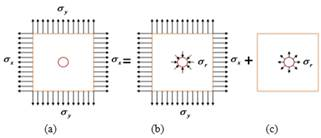Figure 4. Equivalent diagram of the stress on the perforated plate

The principle of coefficient calibration by finite element method is shown in Fig.4. The stress effect in Fig.4 (a) is equivalent to the superposed stress effect in Fig.4 (b) and Fig.4 (c). In Fig.4 (b), it is considered that there is no displacement deformation, so the displacement deformation in Fig.4 (a) is equivalent to the displacement deformation in Fig.4 (c). At last, the stress effect in Fig.4 (c) was used in model to calibrate the coefficients.

The finite element method is a powerful tool to solve engineering problems [11, 12]. In the simulation, a 2D model is build, which is shown in Fig.5, and the mesh style was CAX4R. The radius of the hole was=1 mm, and the depth of the hole was 1.89 mm, and the particular point, which was 1.2 r away from the center of the hole, was used to calculate the coefficient, and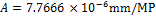a.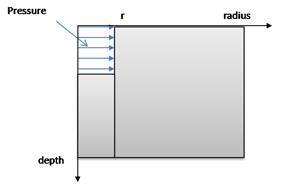Figure 5. Model of the interference fit specimen

5. Results and Discussion

Assuming the sleeve and the plate are the same material, and theoretically, the residual stress in the plate is axisymmetric, which is given by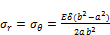(4)

where a and b represent the radius of the plate and the outer radius of the sleeve after assemble, respectively, d  represents the magnitude of interference. And, the theoretical stress magnitude is calculated by Eq.(4)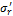=-88.82MPa.

After the coefficient calculation, the stress is calculated by taking the radial displacement of the particular point into Eq.(2), and the experimental stress of every point on the plate is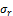=-80.83MPa. The experiment result is smaller when compared to the theoretical value, and the experimental error is 9%, which may be caused by that: the external mechanical pressures are not uniform when the interference fit specimen was assembled.

The interference fit specimen is widely used in mechanical and manufacture process, and in this paper, the result provides the experimental data of the residual stress’s distribution of the interference fit specimen, and lays a foundation for applications of the interference fit specimen.

6. Conclusions

In this paper, the moiré interferometry and hole-drilling method was used to measure the residual stress of the interference fit specimen. Finite element method was applied to calculate the coefficients, and the formula between stress and displacement was obtained. A good agreement between the experimental result and the result is achieved, which manifests that the moiré interferometry and hole-drilling method can measure the residual stress effectively.

Acknowledgements

The authors are grateful to the financial support by the National Natural Science Foundation of China (Grant Nos. 11232008 and 11372118).

References

1. A. S. Franchim, V.S. de Camposa, D. N. Travessa, and C.D. Moura Neto, "Analytical modelling for residual stresses produced by shot peening". MATER DESIGN, 2009. 30(5): p. 1556-1560
2. S.M.H Gangaraj, M. Guagliano, and G.H. Farrahi, "An approach to relate shot peening finite element simulation to the actual coverage". SURF COAT TECH, 2014. 243: p. 39-45.
3. T.Hong, J.Y. Ooi, and B. Shaw, "A numerical simulation to relate the shot peening parameters to the induced residual stresses". ENG FAIL ANAL, 2008. 15(8): p. 1097-1110.
4. P.J. Withers and H. Bhadeshia, "Overview - Residual stress part 1 - Measurement techniques". MATER SCI TECH-LOND, 2001. 17(4): p. 355-365.
5. B. Zuccarello and G. Di Franco, "Numerical-experimental Method for the Analysis of Residual Stresses in Cold-expanded Holes". EXP MECH, 2013. 53(4): p. 673-686.
6. M. Frija, T. Hassine, R. Fathallah, C. Bouraoui, and A. Dogui,"Finite element modelling of shot peening process: Prediction of the compressive residual stresses, the plastic deformations and the surface integrity". MAT SCI ENG A-STRUCT, 2006. 426(1-2): p. 173-180.
7. N.S. Rossini, M. Dassisti, K.Y. Benyounis, and A.G. Olabi, "Methods of measuring residual stresses in components". MATER DESIGN, 2012. 35: p. 572-588.
8. P.J. Withers, "Residual stress and its role in failure". REP PROG PHYS, 2007. 70(12): p. 2211-2264
9. H. Schmidt and J. Hattel, "A local model for the thermomechanical conditions in friction stir welding". MODEL SIMUL MATER SC, 2005. 13(1): p. 77-93.
10. H.M. Xie, B. Li, R. Geer, B. Xu, and J. Castracane, "Focused ion beam Moire method". OPT LASER ENG, 2003. 40(3): p. 163-177.
11. T. Kim, H. Lee, M. Kim, and S. Jung," A 3D FE model for evaluation of peening residual stress under angled multi-shot impacts". SURF COAT TECH, 2012. 206(19-20): p. 3981-3988
12. F. Yang, Z. Chen, and S.A. Meguid, "3D FE modeling of oblique shot peening using a new periodic cell". INT J MECH MATER DES, 2014. 10(2): p. 133-144

 Contents 1. 2. 3. 4. 5. 6.
Article ToolsAbstractPDF(741K)